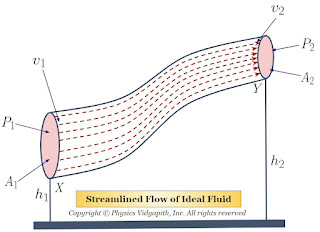## High energy physics

What is high energy physics?

High energy physics, also known as particle physics, is a branch of physics that studies the behavior and properties of subatomic particles at high energies. It is concerned with the fundamental constituents of matter and the interactions between them.

High energy physics involves the use of particle accelerators and detectors to create and study high-energy particles. These accelerators accelerate particles to very high speeds, close to the speed of light, and smash them into each other or a target, creating new particles that can be studied.

The study of high energy physics has led to many important discoveries, such as the existence of quarks and leptons as fundamental particles, the discovery of the Higgs boson, and the discovery of neutrino oscillations. It is also relevant to understanding the nature of the universe at its most fundamental level, including the origin of the universe, the nature of dark matter, and the behavior of high-energy cosmic rays.

## Popular Posts

### Bernoulli's Theorem and Derivation of Bernoulli's EquationStatement of Bernoulli's Theorem: When an ideal fluid (i.e incompressible and non-viscous Liquid or Gas) flows in streamlined motion from one place to another, then the total energy per unit volume (i.e Pressure energy + Kinetic Energy + Potential Energy) at each and every of its path is constant. $P+\frac{1}{2}\rho v^{2} + \rho gh= constant$ Derivation of Bernoulli's Theorem Equation: Let us consider that an incompressible and non-viscous liquid is flowing in streamlined motion through a tube $XY$ of the non-uniform cross-section. Now Consider: The Area of cross-section $X$ = $A_{1}$ The Area of cross-section $Y$ = $A_{2}$ The velocity per second (i.e. equal to distance) of fluid at cross-section $X$ = $v_{1}$ The velocity per second (i.e. equal to distance) of fluid at cross-section $Y$ = $v_{2}$ The Pressure of fluid at cross-section $X$ = $P_{1}$ The Pressure of fluid at cross-section $Y$ = $P_{2}$ The height of cross-section $X$ from